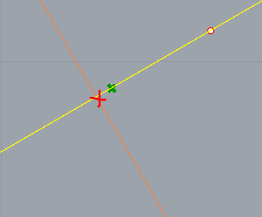# Basics - Angles between two vectors and (IF THEN ELSE) regrouping

Hi all,

two basic questions in one thread.

I have a 3D polyline (road red line) to which I would like to measure the angles to the vector projected on the horizontal plane (basically the road inclination). I managed to do so but :

1. I cannot find positive and negative values. The work around I found was to define the origin of the projection to 0,0,0. In this case I have small and big numbers and I can spot where the inclinaison because negative (downwards) If (alpha > 180) then (alpha -360).

2. Following the method above how to regroup in a list the the result of this operation and the else condition (keep alpha if the above answer if false)? Basically it shall do a list as that one of an basic Excel file IF (condition, if true [Y], if false [leave X]). Sort of something like the “pick and choose” but allowing for different amount of items in the 0 and 1 input…

I know this is very basic. Sorry for that in advance I’m beginning discovering this GH world…

Angle and IF question.gh (14.5 KB)
Angle and IF question.3dm (179.9 KB)

Hello,

The `Angle` component always retrieves the smallest angle, in absolute value, between two vectors, unless you provide it a plane that acts as a polar system to do an oriented measure. If so, the angle is measures from Vestor A to Vector B, both projected on the plane, going anti-clockwise around the Z axis of the plane.

I changed your global plane to a local vertical plane constructed at each point from the tangent to the curve and the world Z axis. However this still gives values between 0 and 2Pi, a `Pick'n'Choose` is then needed to do the if/then/else procedure that leads to an angle between -Pi and Pi.

I’ve added extra examples if you want to only retrieve positive joints, or do something else based on this criteria.

Have a look at this also If statement on empty branch - #4 by magicteddy

Angle and IF question.gh (17.1 KB)

1 Like

Thank you once again @magicteddyYes your plane definition is definitely simpler - I tried with the `Plane 3pt` to begin with but could manage to center it at the intersection point with correct results.
If I understand you correctly, despite the plane definition there is no way to retrieve directly positive and negative results, isn’t? And thank you for the explication of the `Pick'n'Choose` component …

Side question : what if instead of a single curve I has multiple poly lines - how comes in your opinion that the intersection issued from the closest point is not overlapping the orange joint lines (they are constructed to be totally perpendicular to the red line hence the perpendicular should be the closest point indeed - but I’m obviously missing something out…)?Cheers.

Angle and IF question.3dm (318.1 KB)
Angle and IF question - ANSWER.gh (35.6 KB)

1 Like

Here’s another method to get your road inclination angles with positive and negative values:

Angle and IF question_221222a.gh (37.8 KB) - Geometry internalized from your file.

-Kevin

@grasping_GR The closest point retrieves the point closest to the curve, going perpendicular to it, so because of the slope, there is a horizontal offset. You were right to use `Surface | Curve`.

@kev.r Nice sneaky trick using polar coordinates, I’d never have thought about it ! Thanks. I’m not a fond of the barbarian flattening, but I can live with it